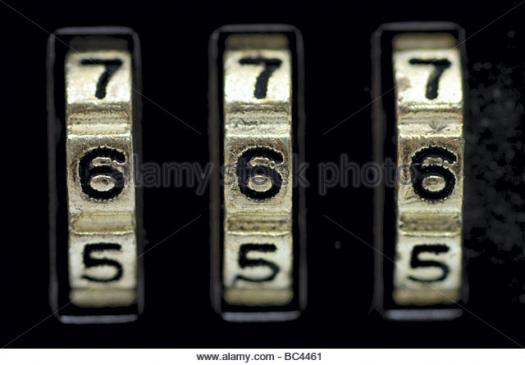# What Do You Know About One-step Equations Intuition?

Approved & Edited by ProProfs Editorial Team
The editorial team at ProProfs Quizzes consists of a select group of subject experts, trivia writers, and quiz masters who have authored over 10,000 quizzes taken by more than 100 million users. This team includes our in-house seasoned quiz moderators and subject matter experts. Our editorial experts, spread across the world, are rigorously trained using our comprehensive guidelines to ensure that you receive the highest quality quizzes.
| By Livyn
L
Livyn
Community Contributor
Quizzes Created: 347 | Total Attempts: 144,443
Questions: 10 | Attempts: 123SettingsIn mathematics, an equation is a statement of an equality containing one or more variables. Solving the equation consists of determining which values of the variables make the equality true.

• 1.

### Equations is a topic under ....?

• A.

Mathematics

• B.

Literature

• C.

Language

• D.

Music

A. Mathematics
Explanation
Equations is a topic that falls under the subject of Mathematics. In Mathematics, equations are used to represent relationships between variables and solve for unknown values. It involves the use of symbols, numbers, and operations to express mathematical relationships and find solutions. Therefore, the correct answer is Mathematics.

Rate this question:

• 2.

### An equation is a statement of an equality containing one or more .....

• A.

Ratios

• B.

Decimals

• C.

Variables

• D.

Proportions

C. Variables
Explanation
An equation is a statement of equality that includes variables. Variables are symbols that represent unknown quantities and can take on different values. In an equation, these variables are typically combined with constants and mathematical operations to express a relationship between different quantities. By solving the equation, we can determine the values of the variables that satisfy the equality. Ratios, decimals, and proportions are not necessarily present in every equation, but variables are a fundamental component.

Rate this question:

• 3.

### Variables are also called what?

• A.

Unknown

• B.

Blank

• C.

Zero

• D.

None

A. Unknown
Explanation
Variables are also called "unknown" because they represent unknown values or quantities that can change in a mathematical equation or problem. They are used to symbolize and manipulate unknown values in order to solve equations and find solutions.

Rate this question:

• 4.

### Variables are also called unknowns and the values of the unknowns which satisfy the equality are called ..... of the equation.

• A.

• B.

Solutions

• C.

Results

• D.

Points

B. Solutions
Explanation
When solving an equation, variables represent unknown quantities. The values of these variables that make the equation true are called solutions. Therefore, the correct answer is "solutions."

Rate this question:

• 5.

### There are how many kinds of equations?

• A.

One

• B.

Two

• C.

Three

• D.

Four

B. Two
Explanation
The question asks about the number of kinds of equations. The given options are one, two, three, and four. The correct answer is two. This implies that there are two different types or categories of equations.

Rate this question:

• 6.

### Mathematics can be taught in ....?

• A.

School

• B.

Home

• C.

Restaurant

• D.

Museum

A. School
Explanation
Mathematics can be taught in school because it is a subject that requires structured learning and guidance from trained teachers. Schools provide a suitable environment for students to engage in mathematical activities, interact with peers, and receive proper instruction. Additionally, schools have dedicated classrooms, resources, and curricula specifically designed to teach mathematics effectively. Home, restaurant, and museum settings may not offer the necessary educational infrastructure and support required for comprehensive mathematics education.

Rate this question:

• 7.

### The "=" symbol, which appears in every equation, was invented in the year .....?

• A.

1557

• B.

1555

• C.

1641

• D.

1890

A. 1557
Explanation
The correct answer is 1557. The "=" symbol, also known as the equals sign, was invented in 1557 by Welsh mathematician Robert Recorde. He introduced this symbol in his book "The Whetstone of Witte" as a way to indicate equality in mathematical equations. Prior to this, mathematicians used various phrases and symbols to represent equality, but Recorde's invention of the "=" symbol provided a concise and universally recognized way to express equality in equations.

Rate this question:

• 8.

### The "=" symbol, which appears in every equation, was invented in 1557 by?

• A.

Liam Nesson

• B.

Robert Recorde

• C.

Albert Eisntein

• D.

Isaac Newton

B. Robert Recorde
Explanation
Robert Recorde is the correct answer because he is credited with inventing the "=" symbol in 1557. Recorde was a Welsh mathematician and physician who introduced the symbol in his book "The Whetstone of Witte." Prior to this, equations were often written out in words, making it difficult to express mathematical concepts concisely. Recorde's introduction of the "=" symbol revolutionized mathematics by providing a clear and efficient way to represent equations.

Rate this question:

• 9.

### Who among them is not a mathematician?

• A.

Robert Recorde

• B.

Barack Obama

• C.

Albert Einstein

• D.

Isaac Newton

B. Barack Obama
Explanation
Barack Obama is not a mathematician because he is a politician and not known for any contributions or expertise in the field of mathematics. On the other hand, Robert Recorde, Albert Einstein, and Isaac Newton are all renowned mathematicians who have made significant contributions to the field.

Rate this question:

• 10.

### Mathematics is related to?

• A.

Algebra

• B.

Drama

• C.

Reproduction

• D.

Civil rights

A. Algebra
Explanation
Mathematics is a field of study that deals with numbers, quantities, shapes, and patterns. Algebra is a branch of mathematics that focuses on the manipulation and study of mathematical symbols and the rules for manipulating these symbols. It involves solving equations, simplifying expressions, and analyzing patterns and relationships. Therefore, algebra is directly related to mathematics.

Rate this question:

Related TopicsBack to top